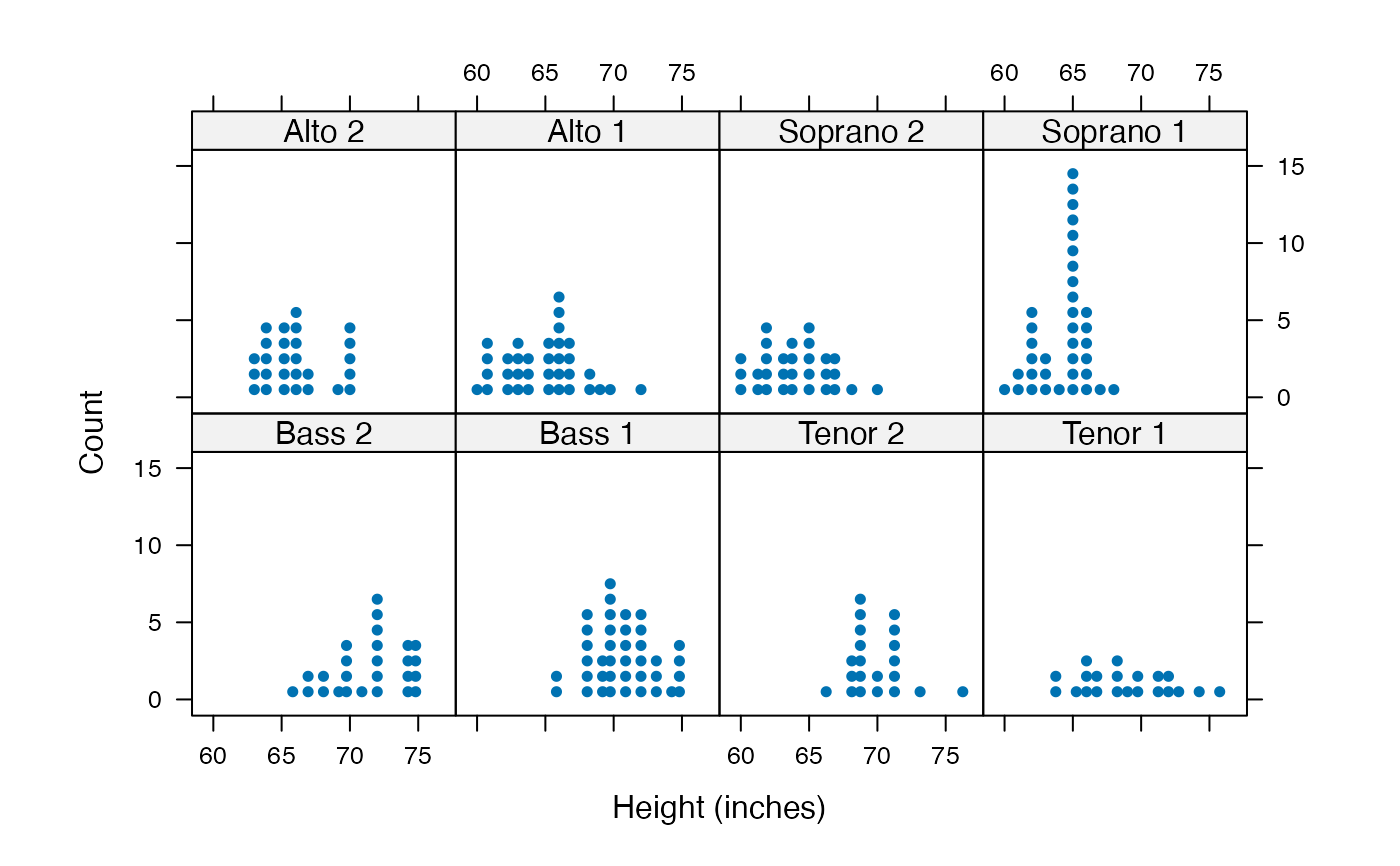A high level function and panel function for producing a variant of a histogram called a dotplot.

dotPlot(x, breaks, ..., panel = panel.dotPlot)

panel.dotPlot(
x,
breaks,
equal.widths = TRUE,
groups = NULL,
nint = if (is.factor(x)) nlevels(x) else round(1.3 * log2(length(x)) + 4),
pch,
col,
lty = trellis.par.get("dot.line")$lty, lwd = trellis.par.get("dot.line")$lwd,
col.line = trellis.par.get("dot.line")$col, alpha = trellis.par.get("dot.symbol")$alpha,
cex = 1,
type = "count",
...
)

## Arguments

x

a vector of values or a formula

breaks, equal.widths, groups, pch, col, lty, lwd, col.line, type, alpha

as in histogram()

...

panel

a panel function

nint

the number of intervals to use

cex

a ratio by which to increase or decrease the dot size

## Value

a trellis object

histogram()

## Examples

if (require(mosaicData)) {
dotPlot( ~ age, data = HELPrct)
dotPlot( ~ age, nint=42, data = HELPrct)
dotPlot( ~ height | voice.part, data = singer, nint = 17,
endpoints = c(59.5, 76.5), layout = c(4,2), aspect = 1,
xlab = "Height (inches)")
}